# HashMap与ConcurrentHashMap实现原理理解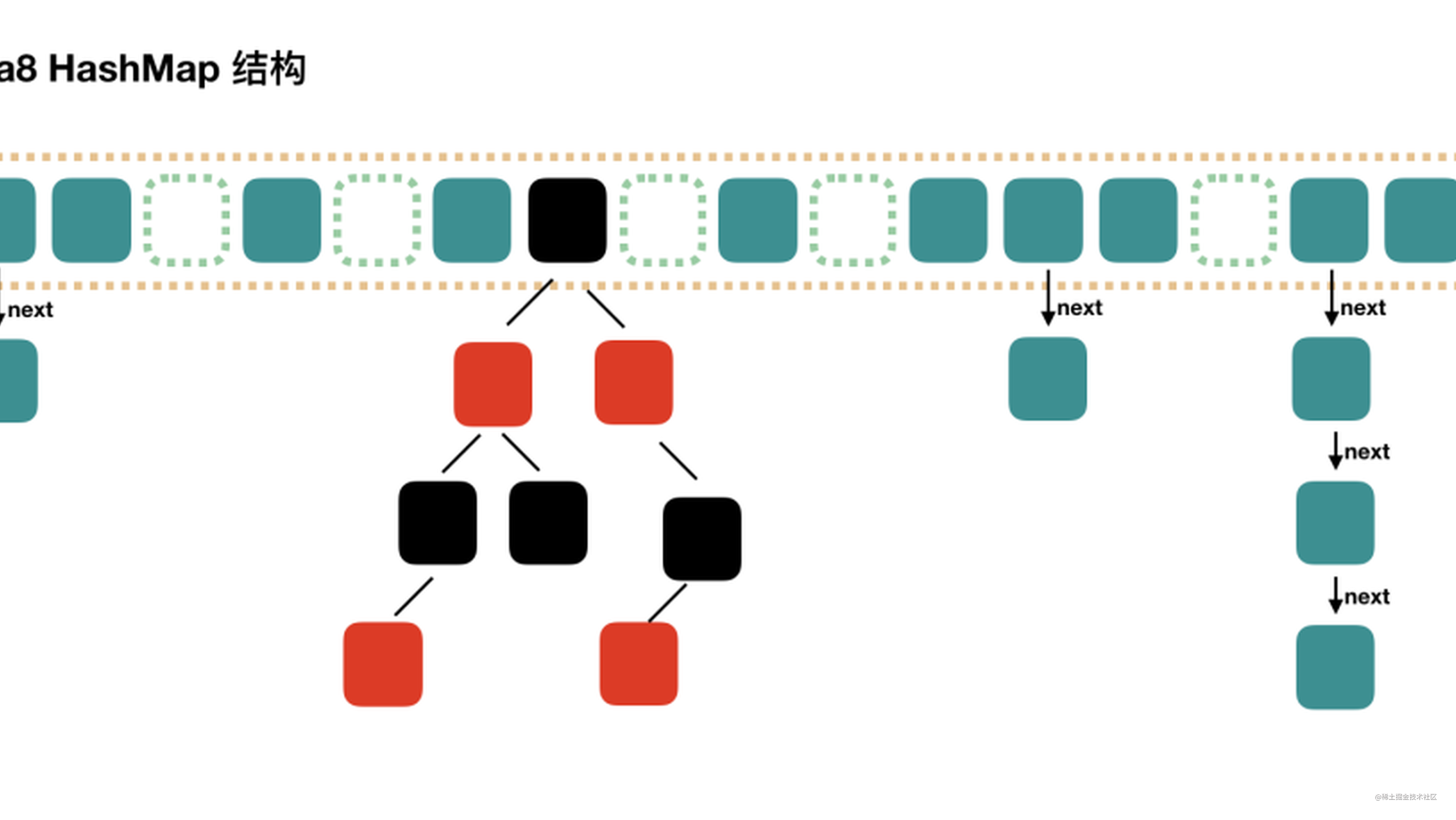Offer 驾到，掘友接招！我正在参与2022春招打卡活动，点击查看活动详情

## HashMap

HashMap是Map家族的骨干成员之一，它继承了AbstractMap抽象类，实现了Map接口，是key-value键值对存储的数据结构，通过键计算hash可以实现快速查找获取值。

HashMap的特性：

• 只能有一个null键，并且null键的下标为0
• key不可以重复
• 是线程不安全的
• 基于数组、链表和红黑树实现的

HashMap在java中的定义:

``````public class HashMap<K,V> extends AbstractMap<K,V>
implements Map<K,V>, Cloneable, Serializable {
}
``````

HashMap的结构图：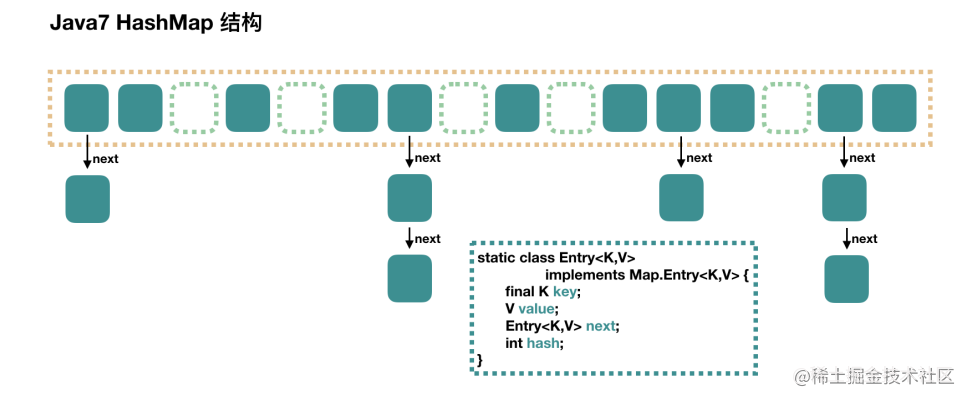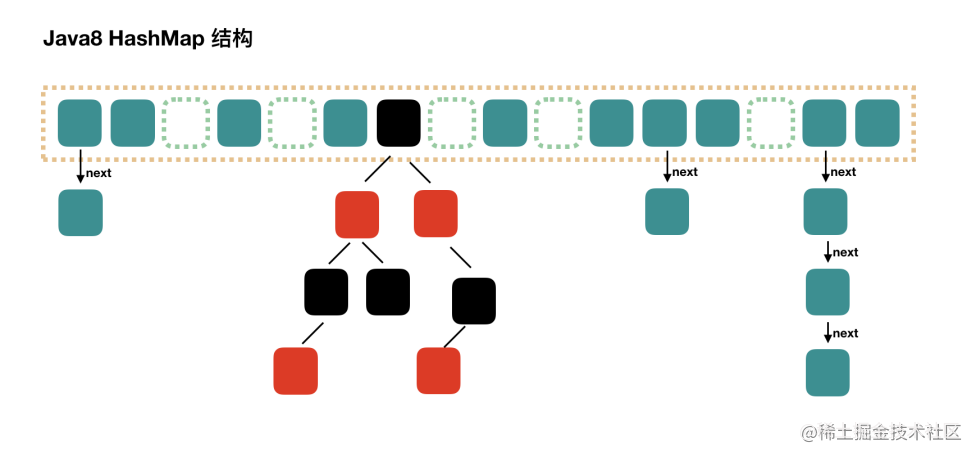JAVA7与JAVA8结构不同的是加入了红黑树，查找的时间复杂度由O(n)变为O(logn)。

#### put方法

HashMap通过put(K key, V value)方法来往集合中存值

``````public V put(K key, V value) {
return putVal(hash(key), key, value, false, true);
}
``````

putVal:

``````final V putVal(int hash, K key, V value, boolean onlyIfAbsent,
boolean evict) {
Node<K,V>[] tab; Node<K,V> p; int n, i;
//如果数组未创建或者数组长度为0，执行resize()方法初始化
if ((tab = table) == null || (n = tab.length) == 0)
n = (tab = resize()).length;
//如果key对应的下标下的节点为null,新建一个节点存入即可
if ((p = tab[i = (n - 1) & hash]) == null)
tab[i] = newNode(hash, key, value, null);
else { //如果hash冲突了
Node<K,V> e; K k;
if (p.hash == hash &&
((k = p.key) == key || (key != null && key.equals(k))))
e = p; //如果hash和key相同，替换掉原来的node,原来的值会被覆盖
else if (p instanceof TreeNode) //如果节点是treeNode节点，执行putTreeVal方法
e = ((TreeNode<K,V>)p).putTreeVal(this, tab, hash, key, value);
else {
for (int binCount = 0; ; ++binCount) {
if ((e = p.next) == null) { //如果结点的下一个节点为null,就把新入节点放到next节点
p.next = newNode(hash, key, value, null);
//如果链表长度大于等于8时，转换为红黑树，但是这里不一定会转换，还有判断数组长度
if (binCount >= TREEIFY_THRESHOLD - 1) // -1 for 1st
treeifyBin(tab, hash);
break;
}
if (e.hash == hash &&
((k = e.key) == key || (key != null && key.equals(k))))
break;
p = e;
}
}
//如果存在key相同的节点
if (e != null) { // existing mapping for key
V oldValue = e.value;
//判断是否开启了不覆盖开关，开启后不能覆盖原值，未开启就设置新值
if (!onlyIfAbsent || oldValue == null)
e.value = value;
afterNodeAccess(e);
return oldValue; //
}
}
++modCount; //操作次数加一
if (++size > threshold) //判断数组里元素是否到达扩容阈值，达到就扩容
resize();
afterNodeInsertion(evict);
return null;
}
``````

#### get方法

get(Object key):通过get方法，传入一个key来获取对应的值

``````public V get(Object key) {
Node<K,V> e;
return (e = getNode(hash(key), key)) == null ? null : e.value;
}
``````

`````` final Node<K,V> getNode(int hash, Object key) {
Node<K,V>[] tab; Node<K,V> first, e; int n; K k;
if ((tab = table) != null && (n = tab.length) > 0 &&
(first = tab[(n - 1) & hash]) != null) {
if (first.hash == hash && // always check first node
((k = first.key) == key || (key != null && key.equals(k))))
return first; //如果通过key找到了对应下标，且下标对应的node的key和要查找的key相同就返回这个节点
if ((e = first.next) != null) {//如果第一个节点没找到，就看看是不是冲突链上
if (first instanceof TreeNode) //如果头节点为treeNode，代表是红黑树，就从树上去查找
return ((TreeNode<K,V>)first).getTreeNode(hash, key);
do {//因为上面已经把first的下一个节点赋值给e了，所以使用do while循环。
if (e.hash == hash &&
((k = e.key) == key || (key != null && key.equals(k))))
return e; //如果在链表中找到了就返回
} while ((e = e.next) != null);
}
}
return null; //都没找到返回null
}
``````

#### hash方法

``````static final int hash(Object key) {
int h;
return (key == null) ? 0 : (h = key.hashCode()) ^ (h >>> 16);
}
``````

null值的hash为0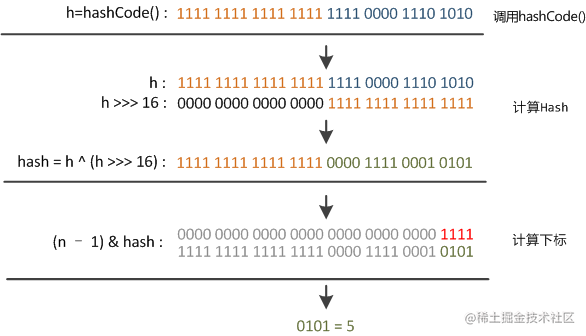#### resize方法

resize方法相对比较复杂一点，主要有以下几点

1. 扩容时计算出新的newCap、newThr，这是两个单词的缩写，一个是Capacity ，另一个是阀Threshold；

2. newCap用于创新的数组桶 new Node[newCap]；

3. 随着扩容后，原来那些因为哈希碰撞，存放成链表和红黑树的元素，都需要进行拆分存放到新的位置中。

#### 扩容时机：

initialCapacity是初始化数组长度，可以调用构造方法传参设置初始化数组大小。

``````static final int tableSizeFor(int cap) {
int n = cap - 1;
n |= n >>> 1;
n |= n >>> 2;
n |= n >>> 4;
n |= n >>> 8;
n |= n >>> 16;
return (n < 0) ? 1 : (n >= MAXIMUM_CAPACITY) ? MAXIMUM_CAPACITY : n + 1;
}
``````

## ConcurrentHashMap

ConcurrentHashMap是java并发编程常使用的容器，它继承了AbstractMap类实现了ConcurrentMap接口，区别于HashMap，它是多线程安全的容器。

``````public class ConcurrentHashMap<K,V> extends AbstractMap<K,V>
implements ConcurrentMap<K,V>, Serializable {
}
``````

• 不允许出现null键和null值，会抛出NullPointerException

• 线程安全的

#### 怎样实现线程安全？

JDK 1.7

ConcurrentHashMap 是一个 Segment 数组，Segment 通过继承ReentrantLock 来进行加锁，所以每次需要加锁的操作锁住的是一个 segment，这样只要保证每个 Segment 是线程安全的，也就实现了全局的线程安全。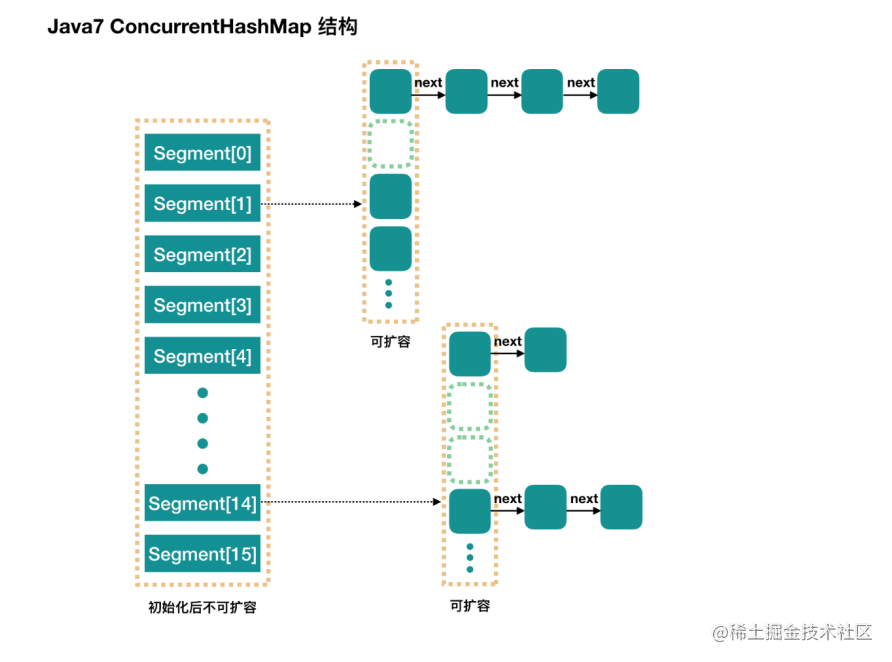JDK 1.8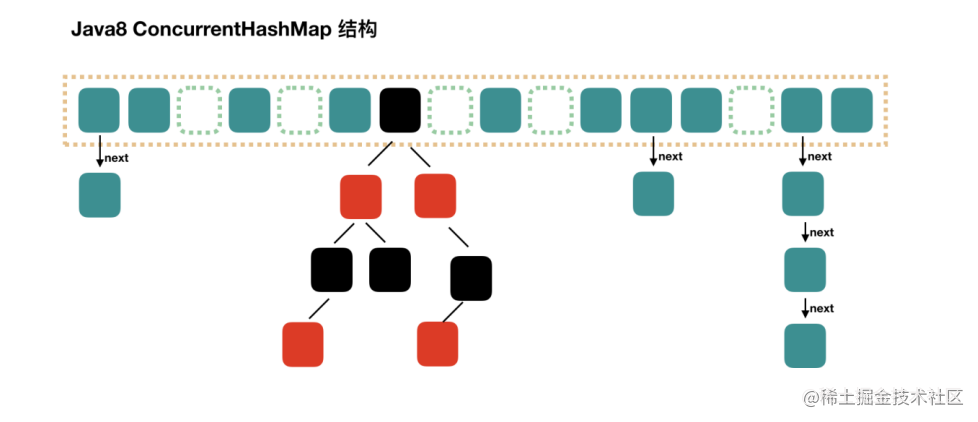ConcurrentHashMap的Node节点与HashMap的节点有些不一样，主要在vaule和next上加了volatile 关键字，用来保持可见性。

``````static class Node<K,V> implements Map.Entry<K,V> {
//链表的数据结构
final int hash;    //key的hash值
final K key;       //key
//val和next都会在扩容时发生变化，所以加上volatile来保持可见性和禁止重排序
volatile V val;   //get操作全程不需要加锁是因为Node的成员val是用volatile修饰
volatile Node<K,V> next;
}
``````

`````` transient volatile Node<K,V>[] table;
``````

``````static final <K,V> Node<K,V> tabAt(Node<K,V>[] tab, int i) {
return (Node<K,V>)U.getObjectVolatile(tab, ((long)i << ASHIFT) + ABASE);
}
``````

#### 线程同步机制：

• 在取得sizeCtl、某个位置的Node的时候，使用的都是unsafe的方法，来达到并发安全的目的

• 当需要在某个位置设置节点的时候，则会通过Synchronized的同步机制来锁定该位置的节点。

• 在数组扩容的时候，则通过处理的步长和fwd节点来达到并发安全的目的，通过设置hash值为MOVED

• 当把某个位置的节点复制到扩张后的table的时候，也通过Synchronized的同步机制来保证现程安全

## 总结

### 参考：

www.zhihu.com/question/20…

blog.csdn.net/weixin_4318…